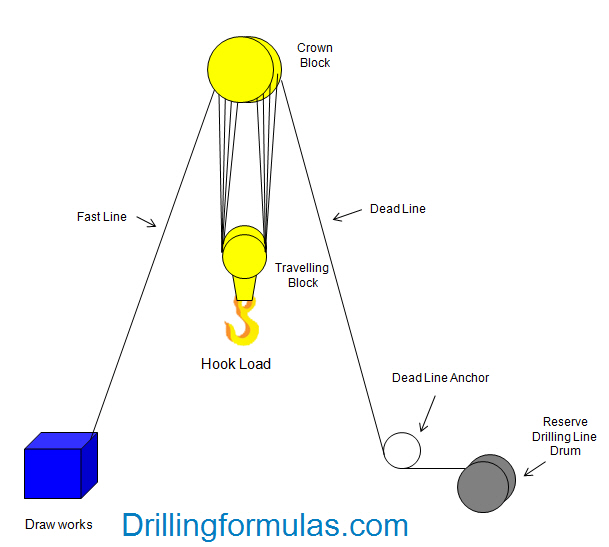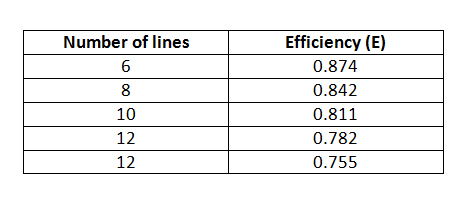The drilling line is reeved over a set of crow block and down to another set of sheaves known as travelling block. The hook connected to travelling block is used to suspend the drilling load. One end of drilling line is wound onto the drawworks and this line is called “Fast Line”. Other end of the drilling line is tied into an anchor point on the rig floor and this line is named as “Dead Line”. The drilling line is reeved around the blocks several times in order to meet required load.According to the diagram above, we can use basic physic to determine derrick load.

Load Analysis Using Free Body Diagram on Travelling Block Under Static ConditionW = N x Tf

Tf = W/N

Where,

N – Number of drilling lines in a travelling block

Tf = Td (Tf and Td are the same value because the same tension in the drilling line)

Load Analysis Using Free Body Diagram on Derrick Under Static ConditionStatic derrick load equates to summation of fast line tension, dead line tension and hook load. We can describe into the following equation.

FD = Tf + W + Td

Where,

Tf – Fast line tension

Note: Neglect a small effect of small angle of the fast line and the dead line.

FD = W/N + W + W/N

FD = (N+2) x W ÷ N

Under dynamic condition, friction in sheave bearings and block lines make the fast tension higher than the dead line tension. It means that the fast line tension will increase under a dynamic condition; however, the dead line tension will remain the same because it is still in static condition.

The fast line tension under the dynamic environments can be described as the equation below;

Tf = W ÷ ( E x N )

Where,

Tf – The fast line tension.

W – Block weight

N – Number of lines

E – EfficiencyTable#1 – Efficiency Factor for Wire Rope Reeving for Multiple Sheave Blocks (API RP9B)

Derrick load under dynamic condition is also equal to summation of hook load, dynamic fast line tension and dead line tension as described in the equation below

FD = Tf + W + Td

Buoyed weight of the drill string is 260,000 lb which will be pulled out of hole. Weight of travelling block and hook is 40,000 lb. The rig has 10 lines strung in crown block and travelling block.

Solution:

Efficiency of 10 lines = 0.811

Total hook load = 260,000 + 40,000 = 300,000 lb

The fast line tension:

Tt = 300,000 ÷ (10 x 0.811)

Tt = 36,991 lb

Td = 300,000 ÷ 10

Td = 30,000 lb

Derrick load under the dynamic condition:

FD = Tf + W + Td

FD = 36,991 + 30,000 + 300,000

FD = 366,991 lb

Derrick load in dynamic condition equates to 366,991 lb

Ref books: Lapeyrouse, N.J., 2002. Formulas and calculations for drilling, production and workover, Boston: Gulf Professional publishing.

Bourgoyne, A.J.T., Chenevert , M.E. & Millheim, K.K., 1986. SPE Textbook Series, Volume 2: Applied Drilling Engineering, Society of Petroleum Engineers.

Mitchell, R.F., Miska, S. & Aadny, B.S., 2011. Fundamentals of drilling engineering, Richardson, TX: Society of Petroleum Engineers.

Share the joyWorking in the oil field and loving to share knowledge.

### 6 Responses to Rig Derrick Load Calculation

1.asim says:

great information for all drilling workers.

2.Girindra Deka says:

now a days everyone use the software developed for drilling calculations. where only thing you need to know is to enter the data correctly. so it is nice to find these calculations which reminds me old days when we learned them.
thanks.

3.Ibrahim Mohammed says:

well done

4.simon says:

this is some good stuff…had I known that this info was provided in the past on this sights for free of cost I would perhaps be more educated …thanks for providing this info

5.Idris Ali says:

useful informations.
thanx

6.riyadh says:

thanks too much for this information.

This site uses Akismet to reduce spam. Learn how your comment data is processed.